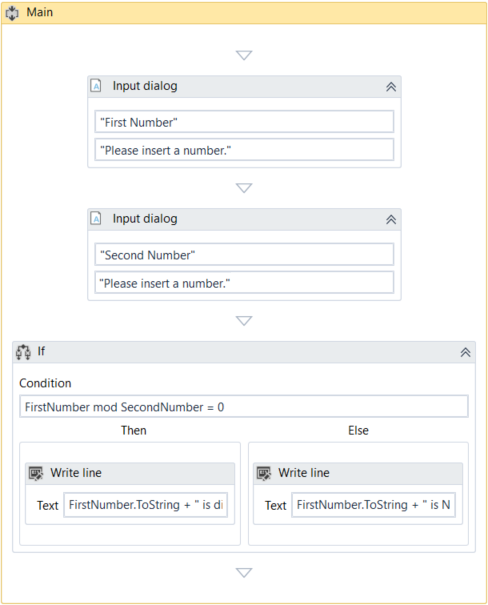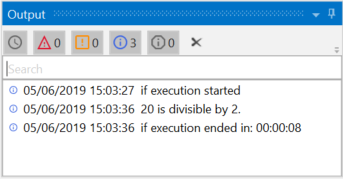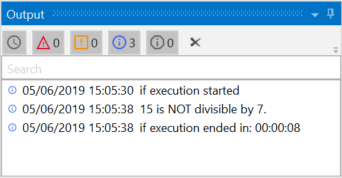Subscribe

# The If Activity

The If activity contains a statement and two conditions. The first condition (the activity in the Then section) is executed if the statement is true, while the second one (the activity in the Else section) is executed if the statement is false.

If activities can be useful to make decisions based on the value of variables.

### 📘Note:

The If activity is almost identical to the Flow Decision one. However, the latter can only be used in flowcharts.

# Example of Using an If Activity

To exemplify how you can use the If activity, let’s create an automation that asks the user for two numbers, checks to see if one is divisible by the other, and depending on the result, displays a different message in the Output panel.

1. Create a new sequence.
2. Create two integer variables, `FirstNumber` and `SecondNumber` for example.
3. Add two Input Dialog activities to the Designer panel.
4. In the Properties panel, type labels and titles for both activities and, in the Result fields, add the `FirstNumber` and `SecondNumber` variables.
5. Add an If activity to the Designer panel, under the previously added Input Dialog ones.
6. In the Condition section, type `FirstNumber` mod `SecondNumber` = 0. This expression checks if the first number is divisible to the second one, using the mod operator.
7. In the Then section, add a Write Line activity.
8. In the Text field, type `FirstNumber.ToString` + " is divisible by "+ `SecondNumber.ToString` + ".". This is the message that is displayed if the first number is divisible by the second one.
9. In the Else section, add another Write Line activity.
10. In the Text field, type `FirstNumber.ToString` + " is NOT divisible by "+ `SecondNumber.ToString` + ".". This is the message that is displayed if the first number is not divisible with the second one.1. Press F5. The automation is executed.
2. Add numbers when prompted. Note that the Output panel displays the result, depending on the values added in the Input Dialog windows.Updated 2 years ago